# CNNs for the Fashion MNIST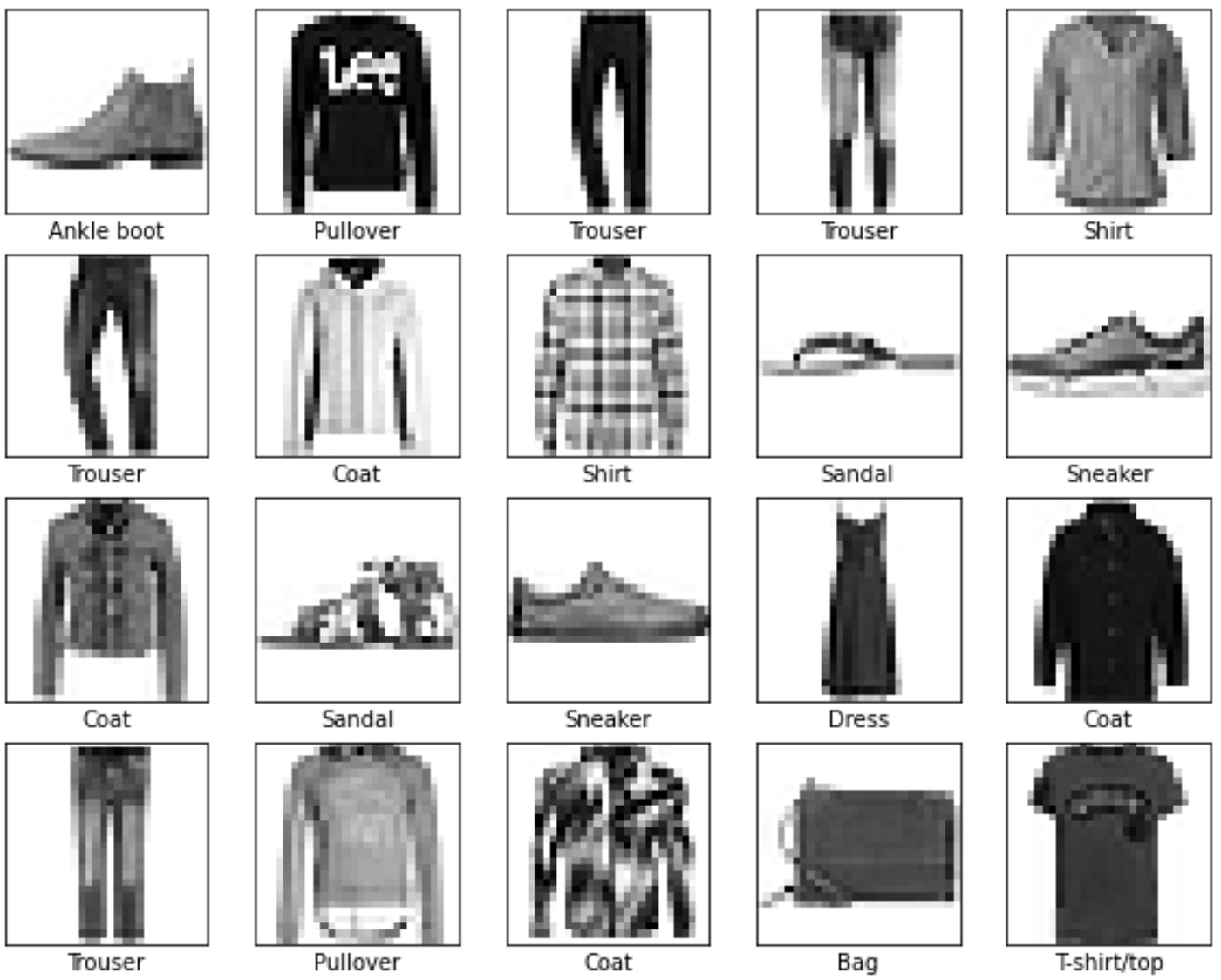The first 20 photos and labels of the Fashion MNIST testing set.

## CNNs for feature recognition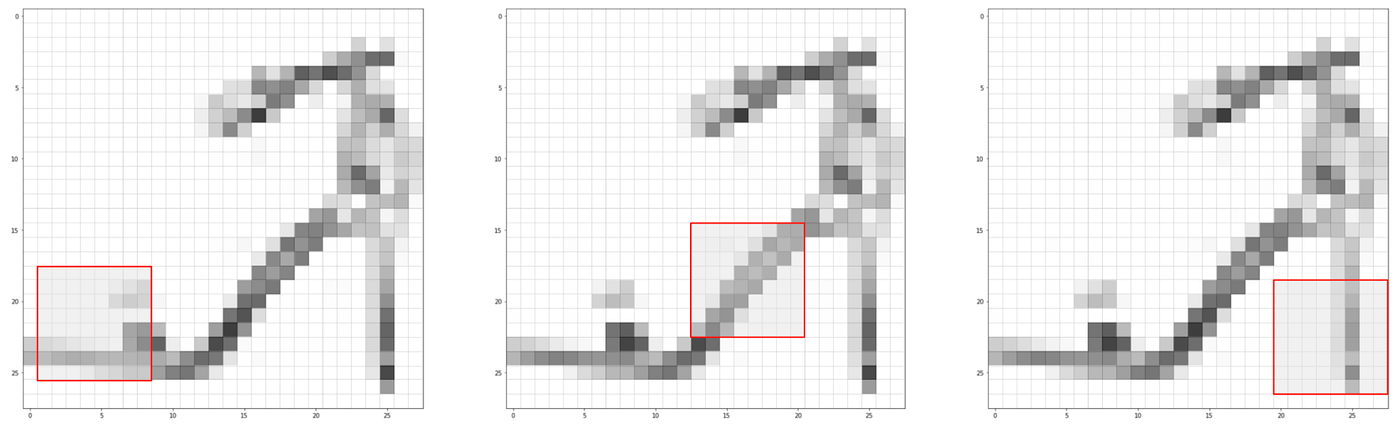The model sees the vamp on the left side, the sole in a diagonal, and the heel on the right side.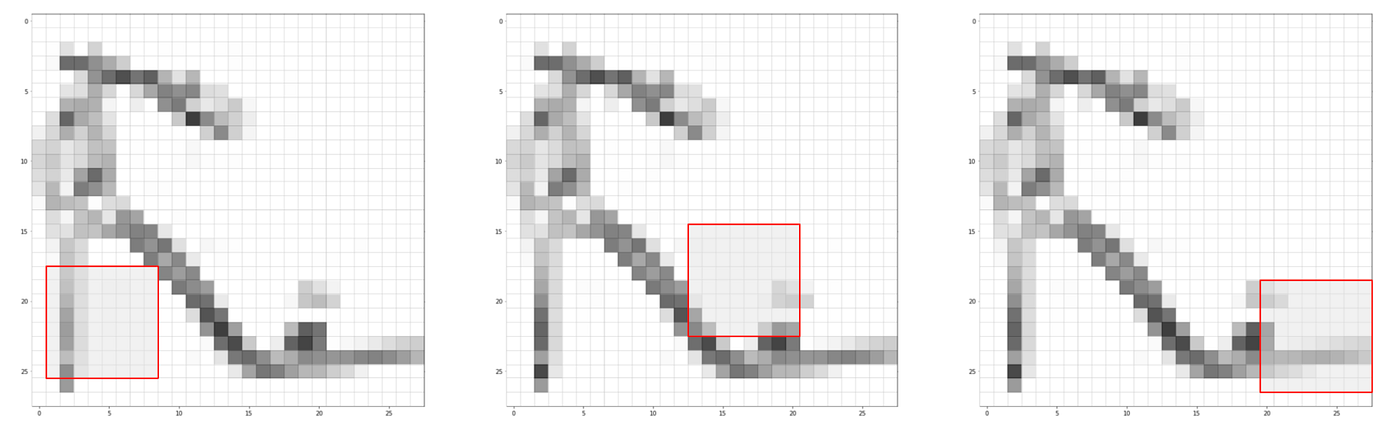The model looks in the same places for the same features, but it won’t find what it’s looking for.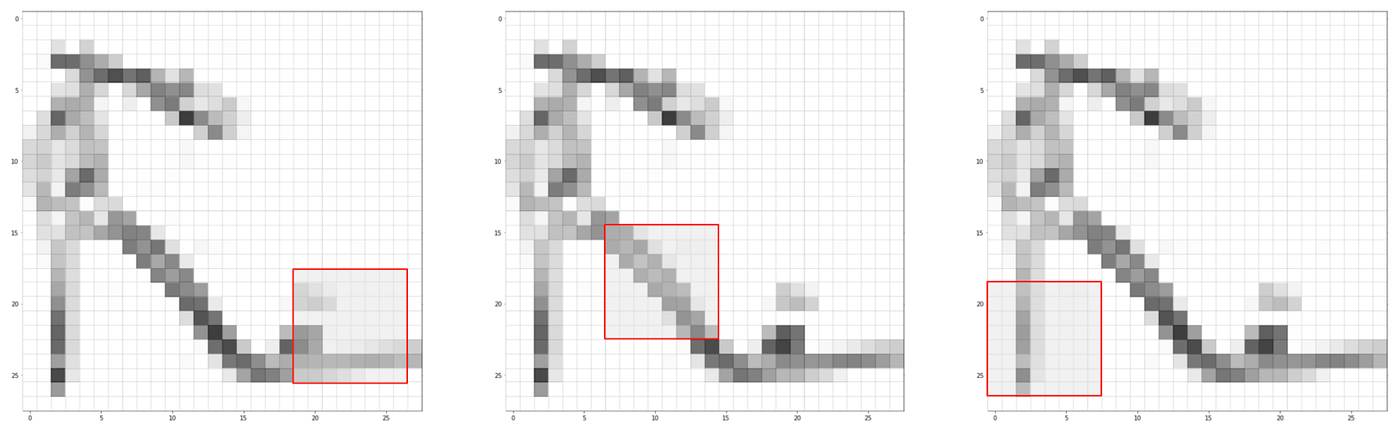The model recognizes the vamp, the sole, and the heel, despite the mixed up order.

## How does the CNN work?

• Filters are small frames through which the model identifies the features. In the case above, the filter is a 7x7 square.
• Strides are what determines how much the filter will move. If your stride length is too small or too large, then the model might identify features too complex or too general.
• Pooling reduces the size of a convolutional layer for more efficient processing. The output is usually the biggest
• Padding is adding extra pixels on the photo border so that the filter covers the entire image. This is useful when a photo cuts off part of a feature, but it’s less relevant in this example as the Fashion MNIST dataset does not cut off any photos.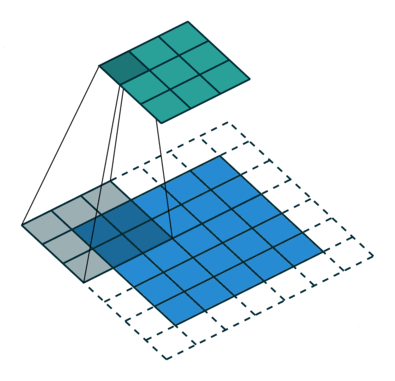This convolution operation has a filter of 3 by 3 with stride length 2 and uses “same” padding. Source.
1. We input the image data as numerical values representing the colour value.
2. The model performs a convolution operation, calculating the dot product of the inputs in a filter.
3. Repeat step 2 each time the model takes a stride and passes a filter over a new area. This creates the feature map.
4. Using pooling, the model reduces the size of the feature map even further.
5. Repeat steps 3–4 at your discretion.
6. We put it through a fully-connected layer for final classification. This is basically the dense neural network we built in part one.

## Building the model

• The number of filters: 32. This corresponds with the number of nodes in this convolutional layer.
• The size of the filter: 3 by 3. The network will identify features that fit within the filter.
• The padding: same. There will be padding on the border to take into account all the pixels.
• The activation function: ReLU. This activation function reduces computing time because it only considers nodes with positive outputs.
• The input shape: 28, 28, 1. The image contains 28 by 28 values, but we’re only considering one colour channel, greyscale. If the images were coloured, it might look like 28, 28, 3. We only need this for the first layer in the network.
`model = models.Sequential()model.add(layers.Conv2D(32, (3, 3), padding='same', activation='relu', input_shape=(28,28,1)))`
`model.add(layers.MaxPooling2D((2,2), strides = 2))`
`model.add(layers.Conv2D(64, (3,3), padding='same', activation = 'relu'))model.add(layers.MaxPooling2D((2,2), strides = 2))`
`model.add(layers.Flatten())model.add(layers.Dense(128, activation = 'relu'))model.add(layers.Dense(10, activation = 'softmax'))`
`train_X = tf.reshape(train_X, [60000, 28, 28, 1])test_X = tf.reshape(test_X, [10000, 28, 28, 1])`

--

--